# 机器学习系列(23)_SVM碎碎念part6：对偶和拉格朗日乘子

## 2.对偶

### 2.1 什么是下界？

S={2,4,8,12}

• 因为1小于等于2，4，8，12，那么1可以是S的下界。

• -3同样符合上面的条件。

• 2是S中的一个元素，同样也是S的一个下界。

### 2.3 我们为什么关心对偶？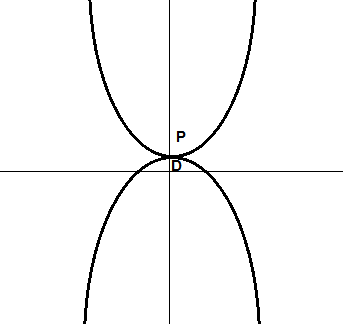## 3.有约束条件的优化问题

### 3.1 注释

minimizexsubjecttof(x)gi(x)=0,i=1,,phi(x)0,i=1,,m

### 3.2 遵守约束是条件什么意思？

minimizexx20

#### 3.2.1 等式约束

minimizexsubjecttox2x=1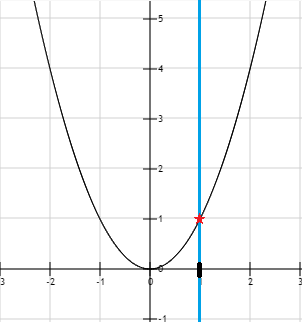x=11

#### 3.2.2 不等式约束

minimizexsubjecttox2x1R={x|x1}

#### 3.2.3 多个约束条件

minimizexsubjecttox2x>=1x<=2minimizexsubjecttox2x=1x<=0

R={x|g(x)=0,h(x)0}

### 3.3 什么是优化问题的解？

minimizexsubjecttof(x)gi(x)=0,i=1,,phi(x)0,i=1,,m

x=inf{f(x)gi(x)=0,i=1,,p,hi(x)0,i=1,,m}

（资源（第二页））

## 4.如何找到有约束优化问题的解?··

### 4.2 等高线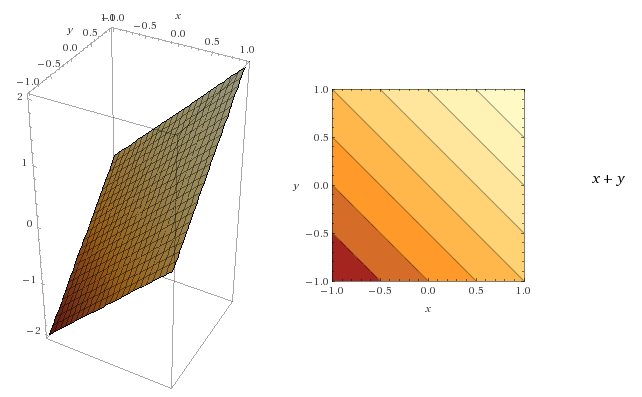• 对线上的任意一点，函数返回相同的值
• 区域颜色越深，函数值越小()

• 垂直于等高线
• 指向函数增加的方向### 4.3 回到拉格朗日乘子法

minimizex,ysubjecttof(x,y)=x2+y2gi(x,y)=x+y1=0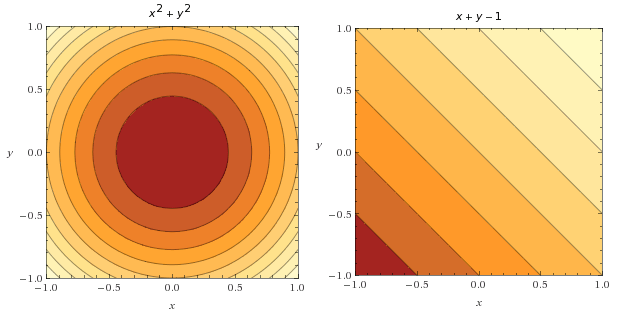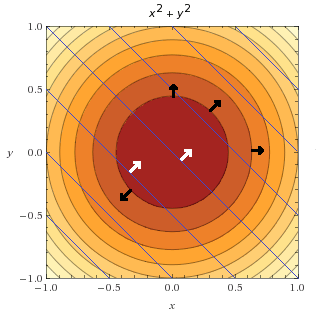x+y1=0x+y=1y=1x#### 4.3.2 如何找到满足 ∇f(x,y)=λ∇g(x,y)$\nabla f(x,y)=\lambda\nabla g(x,y)$ 的点？

f(x,y)λg(x,y)=0$\nabla f(x,y) - \lambda\nabla g(x,y) = 0$

L(x,y,λ)=f(x,y)λg(x,y)$L(x,y,\lambda)=f(x,y)-\lambda g(x,y)$，它的梯度为:

L(x,y,λ)=f(x,y)λg(x,y)$\nabla L(x,y,\lambda)=\nabla f(x,y)-\lambda \nabla g(x,y)$

#### 4.3.3 用拉格朗日乘子法求解例题

minimizex,ysubjecttof(x,y)=x2+y2gi(x,y)=x+y1=0

*第1步：引入拉格朗日函数

L(x,y,λ)=f(x,y)λg(x,y)$L(x,y,\lambda)=f(x,y)-\lambda g(x,y)$

L(x,y,λ)=f(x,y)λg(x,y)$\nabla L(x,y,\lambda)=\nabla f(x,y)-\lambda \nabla g(x,y)$

L(x,y,λ)=0$\nabla L(x,y,\lambda)= 0$

Lx=0Ly=0Lλ=0

2xλ=02yλ=0xy+1=0

2x2y=0

x=y

x$x$ 替换 y$y$ ，代入第三个方程：

xx+1=02x+1=02x=1x=12

12$\frac 1 2$ 替换 x$x$ ，代入第一个方程：

212λ=01λ=0λ=1fx=1/2y=1/2

## 5.结论©️2019 CSDN 皮肤主题: 编程工作室 设计师: CSDN官方博客